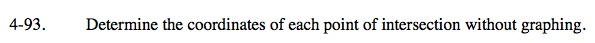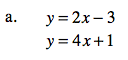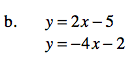### Home > CAAC > Chapter 4 > Lesson 4.2.3 > Problem4-93

4-93.Use the Equal Values Method.

Subtract 2x from both sides.

Subtract 1 from both sides.

Divide by 2 on both sides.

y = y
2x − 3 = 4x + 1

−3 = 2x + 1

−4 = 2x

(−2, −7)Follow the hints and steps used in part (a).

Use the value you found for x and substitute into one of the original equations to solve for y.